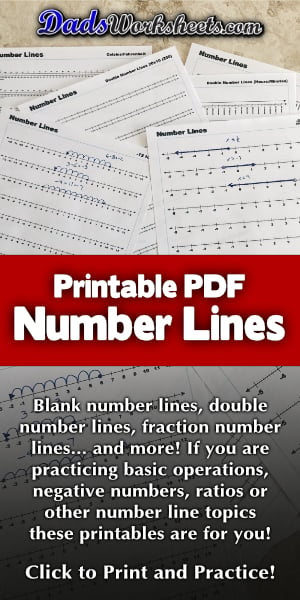# Number Line

A number line can be a powerful tool for learning about negative numbers, ratios or just introductory addition and subtraction operations. The number line PDFs on this page include various ranges (10, 12, 15, 20, 15 and 100) in both starting from zero as well as negative ranges. A complete set of fraction number lines marked with common denominators is included in -5 to 5 ranges. There are also application specific number lines for elapsed time, temperature and money, as well as blank number lines for regular ranges and fractions.

## How to Use a Printable Number Line

A printable number line is a great resource for teaching a wide variety of math topics. Numbers can seem very abstract, and many of the procedures we use to teach math operations are sometimes more formulaic... Getting kids to understand the flow of a sequence of numbers, navigating by adding or subtracting place values or just moving across zero into the realm of negative numbers can be helped greatly with a number line.

The printable number line PDFs on this page are designed for a variety of applications...

### Number Lines to Learn Basic Addition and Subtraction

A number line is a great way for younger grade school students to visualize starting with a number and then moving forward (adding) or backward (subtracting) on the number line to the answer. Much in the same fashion that a multiplication chart can frame the concepts of skip counting and arrays, a number line can be that introduction the the basic linear sequence of numbers. For positive numbers, choose from the 'Number Lines From Zero' and select a value greater than the sum or minuend appropriate for the problem.

As long as your kids are working in the realm of positive numbers, these are the number lines to use, but when you're ready to move into negative numbers, you'll need a number line or two from the next group of printables.

### Number Lines to Learn Negative Numbers

Number lines are a very powerful way to familiarize kids with negative numbers. Often a student's first exposure to negative numbers is when a subtraction problem results in a negative difference. Seeing how a number 'turns negative' when moving left across the zero on a number line is one way to allow students to not only understand what is happening numerically, but to provide an almost mechanical device for kids to self-check their work when dealing with fraction facts. Even when students are comfortable subtracting facts with larger negative answers, the number lines with larger ranges can be useful to check for reasonableness. We usually have one or two of these blank number lines on hand when a negative number unit comes up just to double check a few problems before relying solely on the mechanics of number manipulation in multi-digit subtraction problems.

### Fraction Number Lines to Learn Fraction Operations

Another math topic where a number line can be a powerful conceptual tool is learning fraction addition and fraction subtraction.

The fraction number line printables on this page can help students visualize how to combine both whole numbers and fractional parts using the same concepts and mechanics as navigating integers on a regular number line. And if your kids need help finding equivalent fractions for problems with different denominators, be sure to check out this beautiful fraction chart I created just to help with this tricky step.

### Double Number Lines to Learn Ratios

A double number line is a great way to compare two values and see how they relate to one another. A good example is a problem where a price is given for some quantity of an item, and the student is asked to solve for the price for a different quantity or for the quantity you can purchase with a different amount of money. These are very common word problems, and often students lack a way to visualize the scenario, but by plotting both quantities on a blank double number line students can start to see the relationship between the values and predict where other solutions will occur. a good overview of this technique for solving ratio problems with a double number line is in this Khan Academy video.

### Special Number Lines for Elapsed Time, Money and Temperature Problems

Adding and subtracting values with distinct types of fractional units (for example minutes in an hour or cents in dollars) can be a tricky topic, and a number line marked in time or money units can be a huge help for students struggling with these applied math problems. I've included a few number lines specifically tailored to elapsed time problems and money in this section. Also, I constructed a special double number line with both Fahrenheit and Celsius temperature units which is great for temperature conversion problems, or you can use either scale individually for problems that involve only one measurement system.